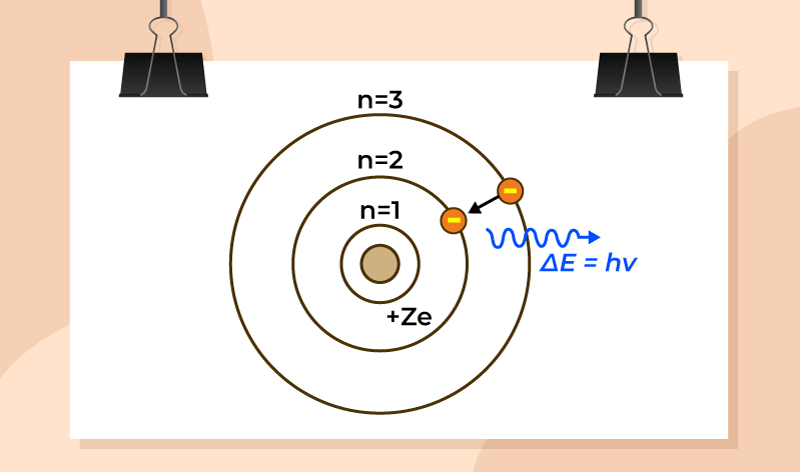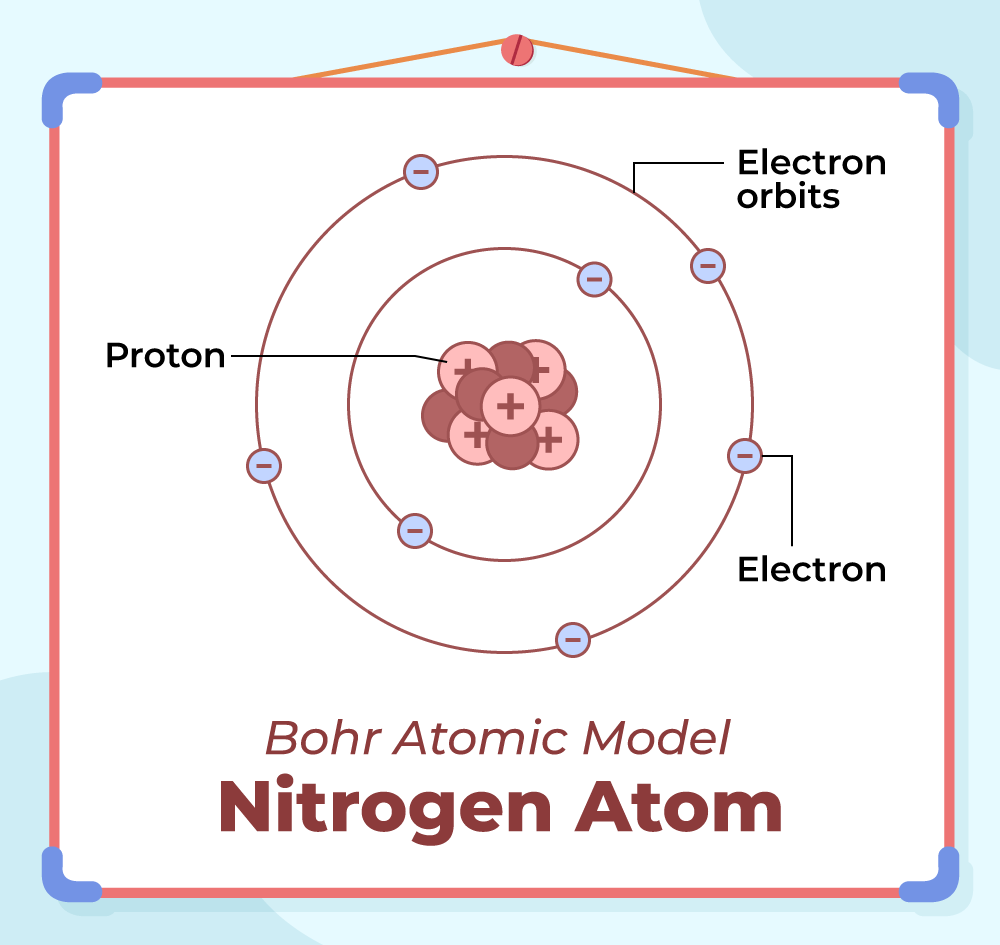GeeksforGeeks App
Open AppBrowser
Continue

# Bohr’s Model of an Atom

Bohr’s Model is an atomic model proposed by Danish Physicist Niels Bohr in 1913. According to this model, in an atom, the electrons revolve around the nucleus in definite energy levels called orbits/shells. This model provides a basic understanding of the concept of the atom and its constituents.

## What is Bohr’s Model of an Atom?

Bohr’s Model of an Atom explains that in an atom all the electrons revolve around the positively charged center called the Nucleus. According to this model, the electron revolving near the nucleus has less energy whereas the electron revolving away from the nucleus has higher energy.

This model proposes that the maximum number of electrons that can be accommodated in any particular orbit is 2n2  where n is the number of orbits. and the maximum number of electrons that can be accommodated in the outermost shell of an atom is 8.

Also, the orbit which is closest to the nucleus has the minimum energy, and the orbit farthest from the nucleus has the maximum energy. Electrons are excited to higher energy levels by absorbing energy and returns to lower energy level by radiating energy.

The energy radiated by the electron while jumping from a higher atomic orbital to a lower atomic orbital is shown in the image below.## History of Bohr’s Atomic Model

Bohr’s model came in the form of an improvement after the previously proposed Atomic models like the cubic model (1902), plum-pudding model (1904), Saturnian model (1904), and Rutherford model (1911). Bohr’s model is mainly the modification based on quantum physics over the Rutherford model, which was based on classical physics.

The model was able to successfully explain the concept of the Rydberg formula for the spectral emission lines of atomic hydrogen. Previously the Rydberg formula was known only experimentally, but theoretically, it was not proved, the Bohr model introduced the theoretical concept successfully.

The previously proposed planetary models of the atom also had some problems. They used to consider electrons spinning in orbit around a nucleus as a charged particle moving in an electric field, but there was no explanation for the fact that the electron would spiral into the nucleus. Viewing in terms of electron emission, this would represent a continuum of frequencies being emitted since, as the electron moved closer to the nucleus, it will move faster and will emit a different frequency which was observed experimentally. These planetary models ultimately predicted all atoms to be unstable due to orbital decay, which was not true, and the phenomenon was successfully explained by Bohr’s model.

## Postulates of Bohr’s Atomic Model

The major postulates of Bohr’s Atomic Model are,

• In an atom, electrons revolve around the nucleus in some fixed circular orbits called shells.
• These shells are also called Stationary Orbits.
• Electrons while revolving in these orbits do not lose any energy. Also, each cell has a fixed energy associated with it.
• In these fixed orbits the angular momentum of the electron is an integral multiple of “h/2π”, where “h” is Planck’s constant.

Therefore the angular momentum “L” of the revolving electron is,

L = nh/2π

where,
n is integer 1,2,3….. known as a principal quantum number
h is Plank’s constant

• The energy of an electron revolving in a stationary orbit remains constant. Energy is emitted from an atom only when an electron jumps from the orbit of higher energy to the orbit having low energy.
• When an electron jumps from one energy level to another energy level it radiates energy. For example, if an electron jumps from orbit with energy E to orbit with energy Ef it releases a photon of energy hv which is calculated as,

hv = Ei – Ef

## Limitations of Bohr’s Atomic Model

The limitations of Bohr’s Atomic Model are,

• Bohr’s Atomic Model does not approve of the Heisenberg Uncertainty Principle.
• Zeeman Effect could not be explained using Bohr’s Atomic Model
• Stark Effect could not be explained using Bohr’s Atomic Model
• Electronic Spectra generated from the large atoms are not explained by Bohr’s Atomic Model

## Application of Bohr Theory

Bohr’s Model is very helpful in explaining the behavior of electrons inside an atom in uni electron species such as Hydrogen which have only 1 electron or hydrogen-like species such as Li+2, Be+3, etc. This model explains the stability of an atom which other models of the atom previous to this failed to explain.

## Bohr Model of Hydrogen Atom

Bohr model of the hydrogen atom was the first atomic model to successfully explain the radiation spectra of atomic hydrogen. Niels Bohr introduced the atomic Hydrogen model in the year 1913. Bohr’s Model of the hydrogen atom attempts to plug in certain gaps as suggested by Rutherford’s model. It holds a special place in history as it gave rise to quantum mechanics by introducing the quantum theory.

### Bohr Model of Nitrogen Atom

Bohr model of the nitrogen atom is explained in the image below it contains seven electrons in its outermost shell.## Distribution of Electrons in Orbits or Shells

Electronic distribution of various orbits or energy levels can be calculated by the formula 2n2. Here, ‘n’ denotes the number of orbits.

The number of electrons in the K shell (1st orbit) can be calculated by 2n2= 2 × 12 = 2. Thus, the maximum number of electrons in 1st orbit = 2

Similarly, The number of electrons in the L shell (2nd orbit)= 2 × 22 = 8. Thus, the maximum number of electrons in the 2nd orbit = 8

The distribution of electrons in the various orbits is discussed in the image below,## Solved Examples on Bohr’s Atomic Model

Example 1: If the velocity of an electron of the first orbit in Bohr’s atomic model of a hydrogen atom is 2.19 × 106 m/s. Find the velocity of electrons in the second orbit.

Solution:

Velocity of an electron in orbit n is given by  vn = v1/n

Given,
v1 = 2.19 × 106 m/s
n = 2

So, v2 = 2.19 × 106 /2

= 1.095 × 106 m/s.

Example 2: The electron in Bohr’s Hydrogen atom, revolves around the nucleus with a speed of 2.18 × 106 m/s in orbit of radius 5.3 × 10-11 m. Find the magnetic field which is produced by electrons on protons in the nucleus.

Solution:

Given,

Radius of orbit, r = 5.3 × 10-11 m

Velocity v= 2.18 × 106 m/s

So, magnetic field, I = Q/T

= e/T

where T = 2πr / v

= (2 × 3.14 × 5.3 × 10-11) / (2.18 × 106)

= 1.528 × 10-16

Therefore,

I = 1.6 × 10-19/ 1.528×10-16

= 1.047 × 10-3 A

Example 3: Find the kinetic energy of an electron in the second Bohr’s orbit of a hydrogen atom.

Solution:

According to Bohr’s postulate

mvr = nh/2π

v = nh/2πmr

K .E = 1/2 mv2

= 1/2 m ( nh / 2πmr ) 2

Example 4: Find the distance between the 2nd and 3rd orbit of Bohr’s atom.

Solution:

Radius of 3rd orbit r3 = 0.529 × n2 × 10-10 / z

= 0.529 × 9 × 10-10

Radius of 2nd orbit r2 = 0.529 × 4 × 10-10

r3 – r2 = 0.529 × (5 × 10 -10)m

= 2.645 A

Example 5: Find the distance between the 2nd and 3rd orbit of Bohr’s atom.

Solution:

Radius of 2nd orbit r2 = 0.529 × n2 × 10-10 / z

= 0.529 × 4 × 10-10

Radius of 1st orbit r1 = 0.529 × 1 × 10-10

r2 – r1  = 0.529 × ( 3 × 10-10) m

= 1.587 A

## FAQs on Bohr Atomic Model

### Q1: What is a Bohr model of an atom?

The model of the atom proposed by famous scientist Neil Bohr in 1915 was came to be known as the Bohr model of an atom. This model was the modification of Rutherford’s model of an atom and states that electron revolves around the nucleus in fixed orbits without losing any energy.

### Q2: What are the postulates of Bohr’s model of an atom?

Major postulates of Bohr’s model of the atom are,

• Electrons revolve around the nucleus in the fixed circular orbits called the shells.
• Electrons do not lose energy when they revolve in these circular orbits(shells)
• They only lose or gain energy if they jump from one orbit to another orbits.
• Each orbit has energy associated to it.

### Q3: What are the limitations of Bohr Atomic Model Theory?

Various limitations of the Bohr Atomic Model are it fails to explain the Zeeman Effect and Stark Effect. It also does not approve the already proven Heisenberg’s Uncertainty Principle.